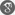##### Cite as:
A. Anguraj, M. Kasthuri, P. Karthikeyan, Integral Boundary Value Problems for Fractional Impulsive Integro Differential Equations in Banach Spaces, Int. J. Anal. Appl., 5 (1) (2014), 56-67.

#### Abstract

We study in this paper,the existence of solutions for fractional integro differential equations with impulsive and integral conditions by using fixed point method. We establish the Sufficient conditions and unique solution for given problem. An Example is also explained to the main results.

#### References

1. A. Anguraj, P. Karthikeyan, and G. M. NGu´er´ekata; Nonlocal Cauchy problem for some fractional abstract integrodifferential equations in Banach space, Communications in Mathematical Analysis , vol.55, no. 6, pp. 1?, 2009.2. A. Anguraj, P. Karthikeyan and J.J. Trujillo; Existence of Solutions to Fractional Mixed Integrodifferential Equations with Nonlocal Initial Condition, Advances in Difference Equations,Volume 2011, Article ID 690653,12pages, doi:10.1155/2011/6906533. B. Ahmad, J. J. Nieto; Existence Results for Nonlinear Boundary Value Problems of Fractional Integrodifferential Equations with Integral Boundary Conditions, Bound. Value Probl.(2009) Art. ID 708576, 11 pp..4. B. Ahmad, A. Alsaedi; Existence of approximate solutions of the forced Duffing equation with discontinuous type integral boundary conditions, Nonlinear Analysis, 10 (2009) 358-367.5. C. Bai; Positive solutions for nonlinear fractional differential equations with coefficient that changes sign Nonlinear Analysis: Theory, Methods and Applications, 64 (2006) 677-685.6. Z. Hu, W. Liu; Solvability for fractional order boundary value problem at resonance, Boundary value problem, 20(2011)1-10.7. J. R Wang, Y. Z. and M. Feckan; On recent developments in the theory of boundary value problems for impulsive fractional differential equations, Computers and mathematics with Applications, 64(2012) 3008-3020.8. A. A. Kilbas, H. M. Srivastava, J. J. Trujillo; Theory and Applications of Fractional Differential Equations, North-Holland Mathematics Studies, 204. Elsevier Science B.V., Amsterdam, 2006.9. V. Lakshmikantham, S. Leela, J. Vasundhara Devi; Theory of Fractional Dynamic Systems, Cambridge Academic Publishers, Cambridge, 2009.10. J. Sabatier, O. P. Agrawal, J. A. T. Machado (Eds.); Advances in Fractional Calculus: Theoretical Developments and Applications in Physics and Engineering, Springer, Dordrecht, 2007.11. S. G. Samko, A. A. Kilbas, O. I. Marichev; Fractional Integrals and Derivatives: Theory and Applications, Gordon and Breach, New York, NY, USA, 1993.12. D. R. Smart; Fixed Point Theorems, Cambridge University Press, 1980.13. X. Su; Boundary value problem for a coupled system of nonlinear fractional differential equations, Applied Mathematics Letters, 22 (2009) 64-69.14. T.L. Guo and W. Jiang, Impulsive problems for fractional differential equations with boundary value conditions, Computers and mathematics with Applications, 64(2012) 3281-3291.15. G. Wang, W. Liu; The existence of solutions for a fractional 2m-point boundary value problems, Journal of Applied Mathematics.16. G. Wang, W. Liu; Existence results for a coupled system of nonlinear fractional 2m-point boundary value problems at resonance, Advances in difference equations,doi:10.1186/1687- 1847-2011-44.17. G.Wang, W. Liu, C. Ren, Existence Of Solutions For Multi-Point Nonlinear Differential Equations Of Fractional Orders With Integral Boundary Conditions , Electronic Journal of Differential Equations, Vol. 2012 (2012), No. 54, pp. 1-10.